# Embedding a trajectory in noise

Motion detection has many functions, one of which is the potential localization of the biomimetic camouflage seen in this video. Can we test this in the lab by using synthetic textures such as MotionClouds?

However, by construction, MotionClouds have no spatial structure and it seems interesting to consider more complex trajectories. Following a previous post, we design a trajectory embedded in noise.

Can you spot the motion ? from left to right or reversed ?

(For a more controlled test, imagine you fixate on the top left corner of each movie.)

(Upper row is coherent, lower row uncoherent / Left is -> Right column is <-)

In :
name = 'trajectory'
%matplotlib inline
import matplotlib.pyplot as plt
import os
import numpy as np
import MotionClouds as mc
fx, fy, ft = mc.get_grids(mc.N_X, mc.N_Y, mc.N_frame)

mc.figpath = '../files/2018-11-13-testing-more-complex'
if not(os.path.isdir(mc.figpath)): os.mkdir(mc.figpath)


Some default parameters for the textons used here:

In :
opts = dict(sf_0=0.04, B_sf=0.02, B_theta=np.inf, B_V=1.1, V_X=2.)


First defining a dense,stationary noise with an horizontal drift:

In :
name_ = name + '_dense'
seed = 42
mc1 = mc.envelope_gabor(fx, fy, ft, **opts)
mc.figures(mc1, name_, seed=seed, figpath=mc.figpath)
mc.in_show_video(name_, figpath=mc.figpath)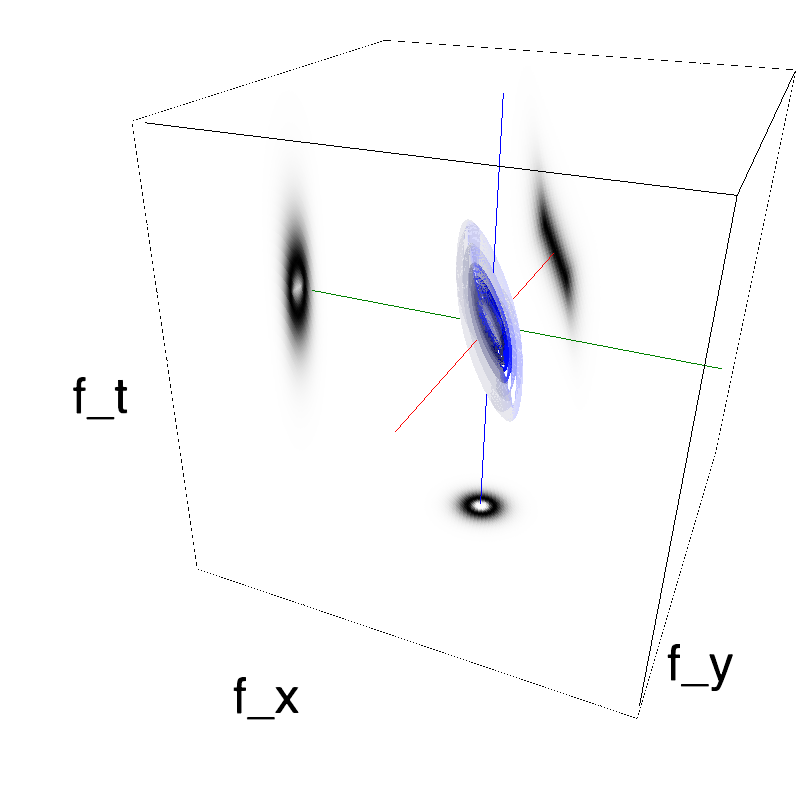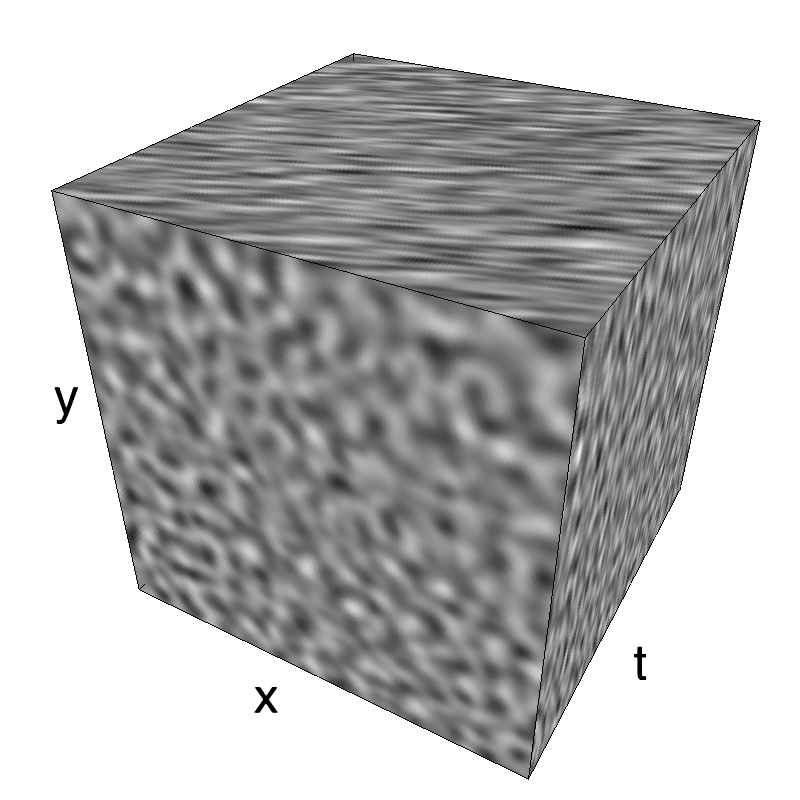The information is distributed densely in space and time.

### one definition of a trajectory¶

It is also possible to show the impulse response ("texton") corresponding to this particular texture (be patient to see a full period):

In :
name_ = name + '_impulse'
seed = 42
mc1 = mc.envelope_gabor(fx, fy, ft, **opts)
mc.figures(mc1, name_, seed=seed, impulse=True, figpath=mc.figpath)
mc.in_show_video(name_, figpath=mc.figpath)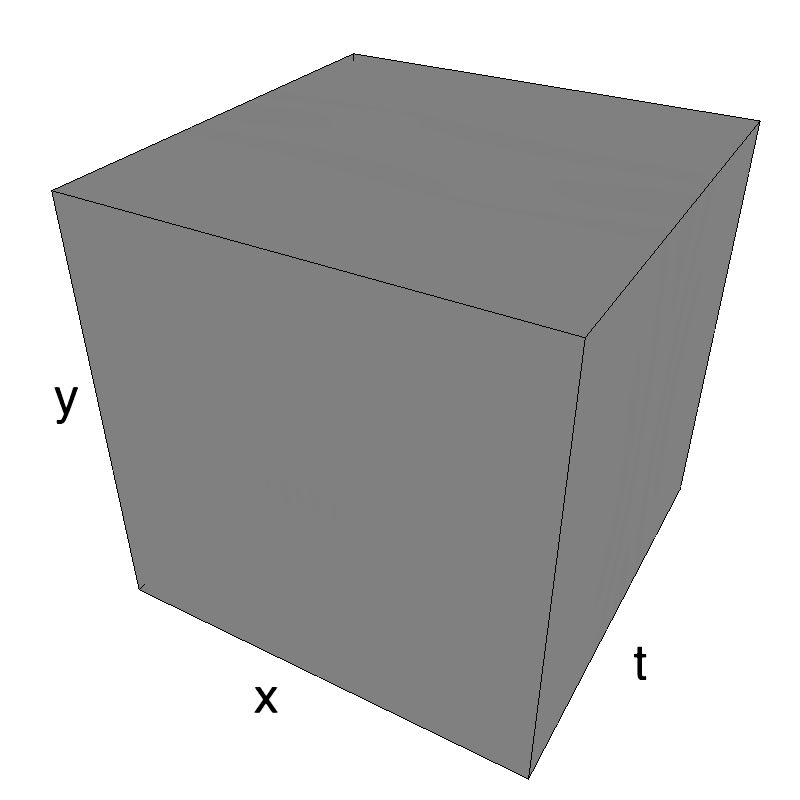To generate a trajectory, we should just convolve this impulse response to a trajectory defined as a binary profile in space and time:

In :
name_ = name + '_straight'
seed = 42
x, y, t = fx+.5, fy+.5, ft+.5 # trick to use fourier coordinates to get spatial coordinates
width_y, width_x = 1/mc.N_X, 1/mc.N_Y
t0, t1 = .375, .625
x0 = .25 #np.random.rand() * (1 - opts['V_X'] * (t1-t0)) # makes sure the trajectory does not wrap across the border
y0 = np.random.rand()
events = 1. * (np.abs(y - y0) < width_y)* (np.abs(x - x0 - opts['V_X']*(t-t0)) < width_x) * (t > t0 ) * (t < t1)

In :
fig, ax = plt.subplots(1, 1, figsize=(15, 15))
ax.imshow(events.mean(axis=1))
ax.set_xlabel('Time')
ax.set_ylabel('X-axis');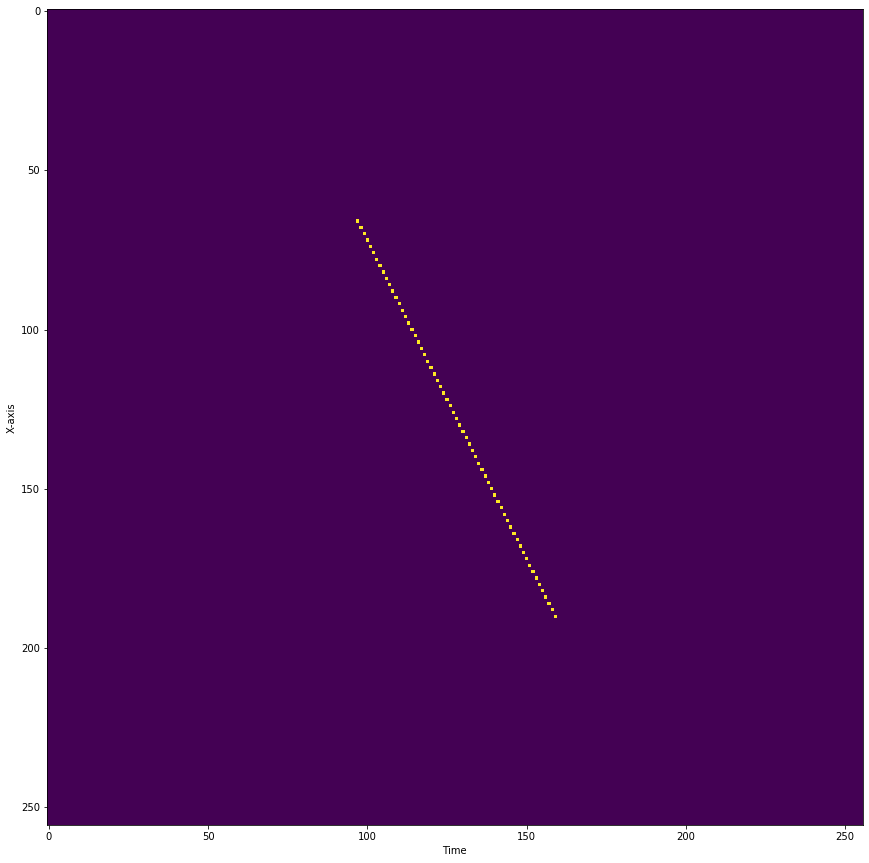In :
mc1 = mc.envelope_gabor(fx, fy, ft, **opts)
mc.figures(mc1, name_, seed=seed, events=events, figpath=mc.figpath)
mc.in_show_video(name_, figpath=mc.figpath)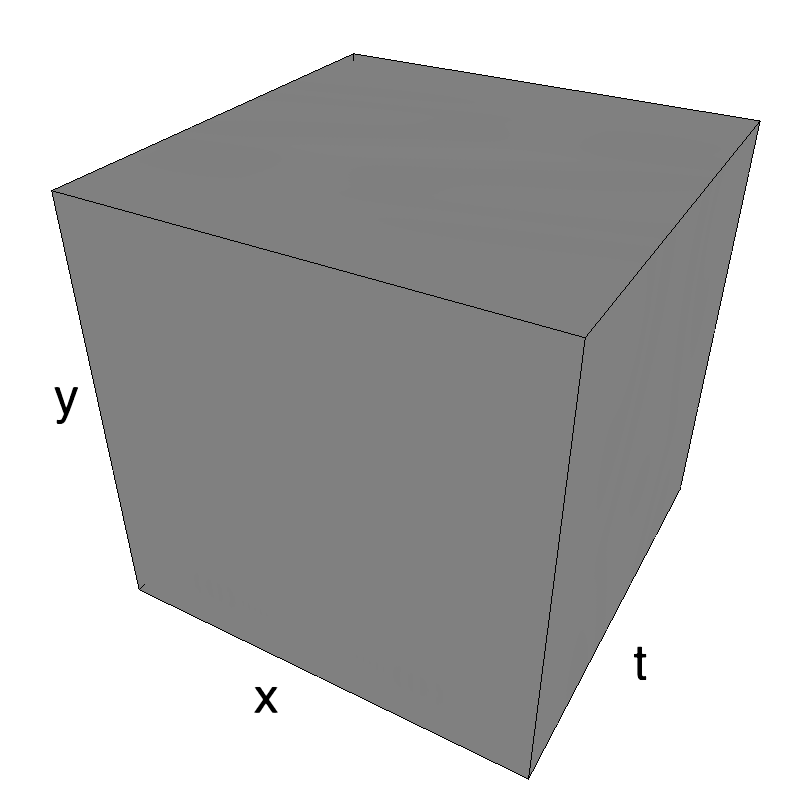To replicate the work from Watamaniuk, McKee and colleagues, we replicate the same motion but where we keep the motion energy while shuffling the coherence:

In :
name_ = name + '_shuffled'
N_folds = 8
N_traj = int((t1-t0)*mc.N_frame) // N_folds
N_start = int(t0*mc.N_frame)
folding = np.random.permutation(N_folds)
events_shuffled = events.copy()
for i_fold in range(N_folds):
S0, S1 = (N_start+folding[i_fold]*N_traj), (N_start+(folding[i_fold]+1)*N_traj)
N0, N1 = (N_start+i_fold*N_traj), (N_start+(i_fold+1)*N_traj)
if False:
nx = int(np.random.rand()*mc.N_Y)
else:
nx = 0 # do not shuffle on the y-axis
ny = int(np.random.rand()*mc.N_Y)
events_shuffled[:, :, S0:S1] = np.roll(np.roll(events[:, :, N0:N1], nx, axis=0), ny, axis=1)

fig, ax = plt.subplots(1, 1, figsize=(15, 15))
ax.imshow(events_shuffled.mean(axis=1))
ax.set_xlabel('Time')
ax.set_ylabel('X-axis');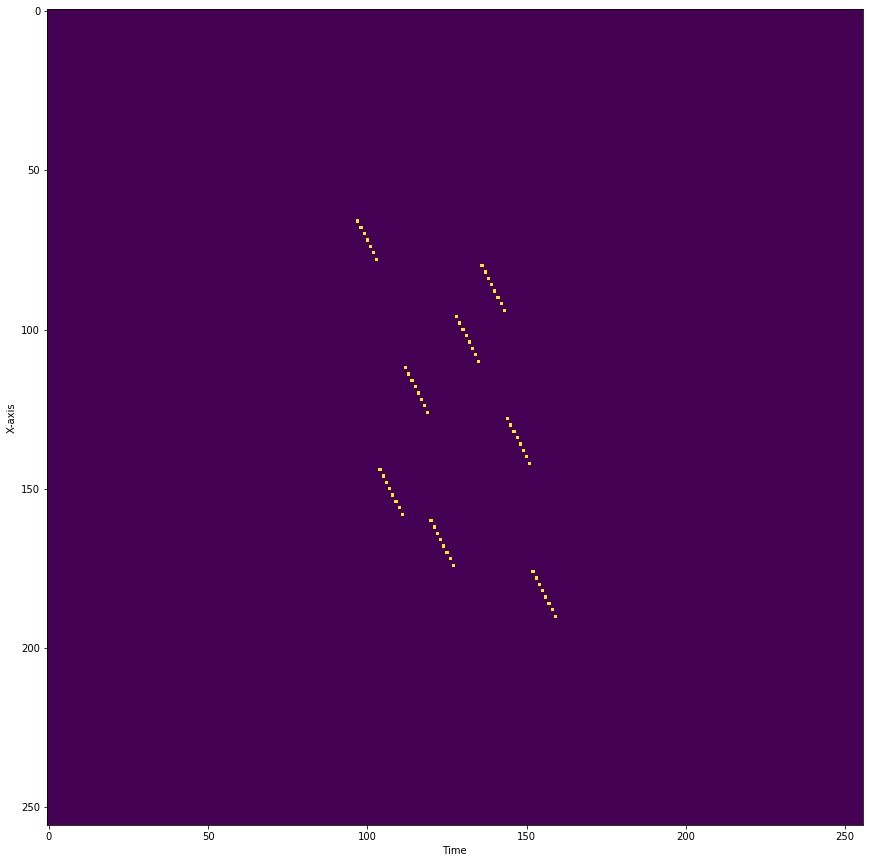In :
mc1 = mc.envelope_gabor(fx, fy, ft, **opts)
mc.figures(mc1, name_, seed=seed, events=events_shuffled, figpath=mc.figpath)
mc.in_show_video(name_, figpath=mc.figpath)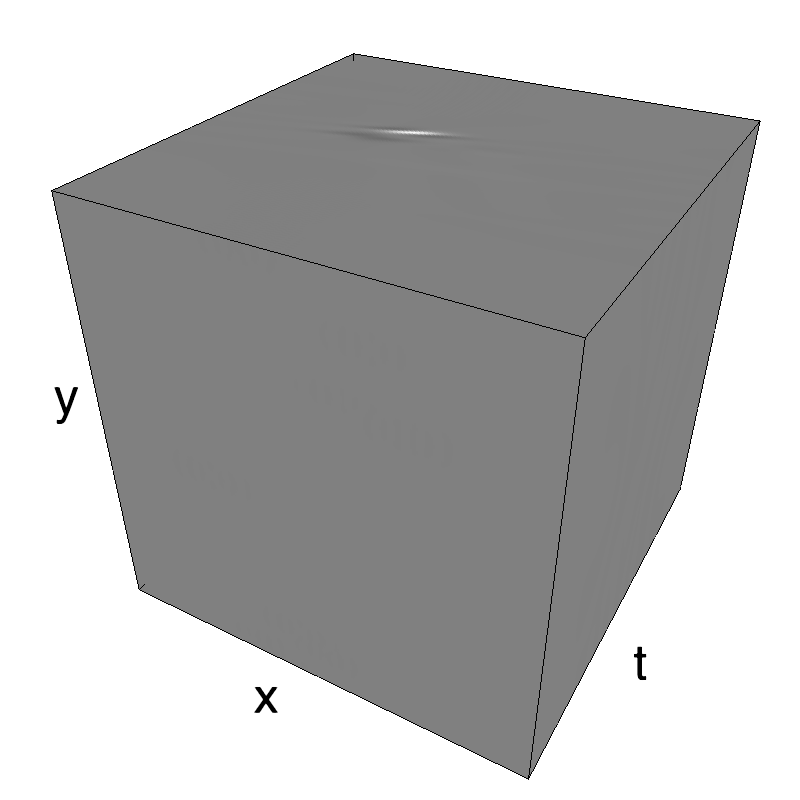### addition of a the trajectory to the incoherent noise¶

It is now possible to add this trajectory to any kind of background, such as a background texture of the same "texton" but with a null average motion:

In :
def overlay(movie1, movie2, rho, do_linear=False):
if do_linear:
return rho*movie1+(1-rho)*movie2
else:
movie1, movie2 = rho*(2.*movie1-1), 2.*movie2-1
movie = np.zeros_like(movie1)
return .5 + .5*movie


a more memory-efficient version of the above:

In :
def overlay(movie1, movie2, rho, do_linear=False):
if do_linear:
return rho*movie1+(1-rho)*movie2
else:
movie1, movie2 = rho*(2.*movie1-1), 2.*movie2-1
movie = movie1 * (np.abs(movie1) > np.abs(movie2)) + movie2 * (np.abs(movie1) <= np.abs(movie2))
#return .5 + .5*movie
return 1. * (movie > 0.)
#seuil = .1
#return .5 + .5 * (-1. * (movie < -seuil) +  1. * (movie > seuil))
#return  .5 + .5* np.tan(4.*movie)

In :
mc1 = mc.envelope_gabor(fx, fy, ft, **opts)
movie_coh = mc.rectif(mc.random_cloud(mc1, seed=seed, events=events))
opts_ = opts.copy()
opts_.update(V_X=0)
mc0 = mc.envelope_gabor(fx, fy, ft, **opts_)
movie_unc = mc.rectif(mc.random_cloud(mc0, seed=seed+1))

In :
name_ = name + '_overlay'
rho_coh = .9
mc.anim_save(overlay(movie_coh, movie_unc, rho_coh), os.path.join(mc.figpath, name_))
mc.in_show_video(name_, figpath=mc.figpath)

In :
name_ = name + '_overlay_difficult'
rho_coh = .5
mc.anim_save(overlay(movie_coh, movie_unc, rho_coh), os.path.join(mc.figpath, name_))
mc.in_show_video(name_, figpath=mc.figpath)


Though it is difficult to find the coherent pattern in a single frame, one detects it thanks to its coherent motion.

In :
name_ = name + '_overlay_shuffled'
movie_coh_shuffled = mc.rectif(mc.random_cloud(mc1, seed=seed, events=events_shuffled))
rho_coh = .9
mc.anim_save(overlay(movie_coh_shuffled, movie_unc, rho_coh), os.path.join(mc.figpath, name_))
mc.in_show_video(name_, figpath=mc.figpath)

In :
name_ = name + '_overlay_difficult_shuffled'
rho_coh = .5
mc.anim_save(overlay(movie_coh_shuffled, movie_unc, rho_coh), os.path.join(mc.figpath, name_))
mc.in_show_video(name_, figpath=mc.figpath)


### trying out different directions¶

In :
rho_coh = .9
name_ = name + '_overlay_reversed'
mc.anim_save(overlay(movie_coh[::-1, :, :], movie_unc, rho_coh), os.path.join(mc.figpath, name_))
mc.in_show_video(name_, figpath=mc.figpath)

In :
name_ = name + '_overlay_shuffled_reversed'
mc.anim_save(overlay(movie_coh_shuffled[::-1, :, :], movie_unc, rho_coh), os.path.join(mc.figpath, name_))
mc.in_show_video(name_, figpath=mc.figpath)


### some book keeping for the notebook¶

In :
%load_ext watermark
%watermark

2020-01-14T12:40:12+01:00

CPython 3.7.6
IPython 7.11.1

compiler   : Clang 11.0.0 (clang-1100.0.33.16)
system     : Darwin
release    : 19.2.0
machine    : x86_64
processor  : i386
CPU cores  : 36
interpreter: 64bit

In :
%load_ext watermark
%watermark -i -h -m -v -p MotionClouds,numpy,pillow,imageio  -r -g -b

The watermark extension is already loaded. To reload it, use:
2020-01-14T12:40:12+01:00

CPython 3.7.6
IPython 7.11.1

MotionClouds 20180606
numpy 1.19.0.dev0+624d34c
pillow not installed
imageio 2.6.1

compiler   : Clang 11.0.0 (clang-1100.0.33.16)
system     : Darwin
release    : 19.2.0
machine    : x86_64
processor  : i386
CPU cores  : 36
interpreter: 64bit
host name  : fortytwo
Git hash   : e1540f7208339f701049845c207075e5fed6ac7b
Git repo   : https://github.com/laurentperrinet/sciblog.git
Git branch : master